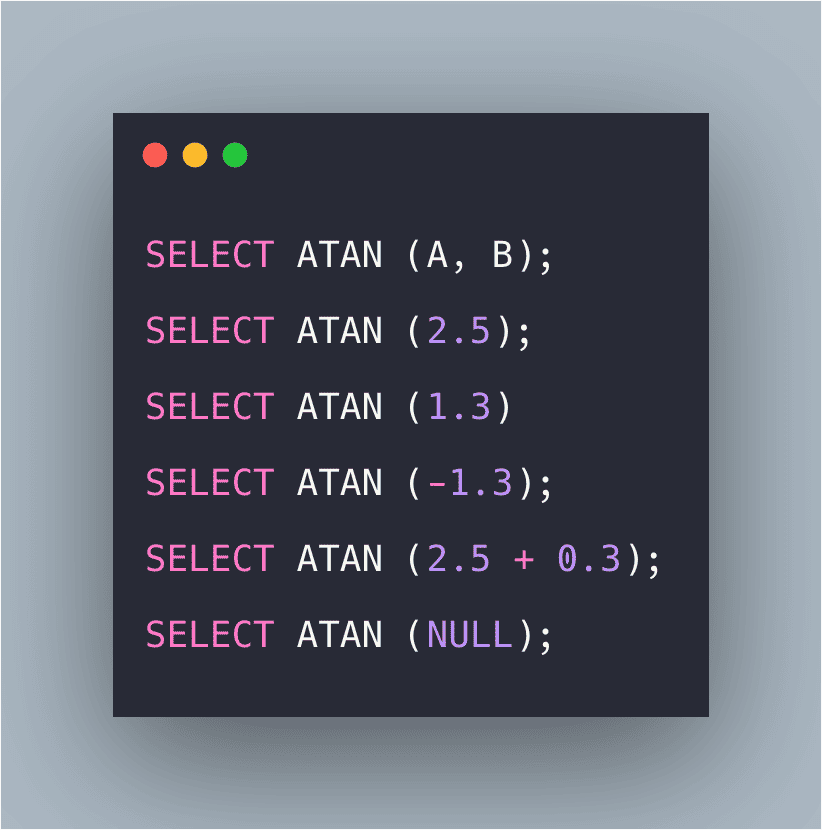# SQL ATAN() Function: The Complete Guide

0
3SQL ATAN() function returns the arctangent of a number.

## SQL ATAN()

SQL ATAN() is a built-in function that is used for finding the arctangent of a number, i.e., it returns angle, in radians, whose tangent is a specified float expression. The SQL ATAN() function returns the arctangent of a value. In other words, it returns the angle, in radians, whose tangent is a specified float expression.

### Syntax

```SELECT ATAN (Expression);

OR

SELECT ATAN (A, B);
```

### Parameters

1. Float_expression: An expression of float or any other type which is implicitly converted to float whose arc tangent value is to be retrieved.
2. A, B: Two values whose arctangent value is to be calculated.

### Examples

#### Query 1

```SELECT ATAN (2.5);
```

#### Output

```1.1902899496825317
```

#### Explanation

Here, the arctangent value is returned.

#### Query 2

`SELECT ATAN (1.3)`

#### Output

`0.91510070055336`

#### Explanation

Here, the arctangent value is returned.

#### Query 3

```SELECT ATAN (-1.3);
```

#### Output

```-0.91510070055336
```

#### Explanation

Here, the arctangent value is returned which is negative.

#### Query 4

```SELECT ATAN (2.5 + 0.3);
```

#### Output

```1.22777238637419
```

#### Explanation

Here, the arc tangent value of the expression is returned.

#### Query 5

```SELECT ATAN (NULL);
```

```NULL
```

#### Explanation

Passing of NULL value as an argument will return a NULL value.